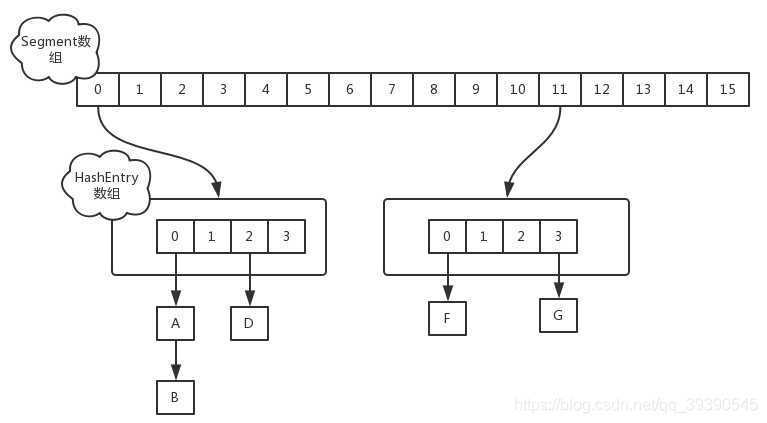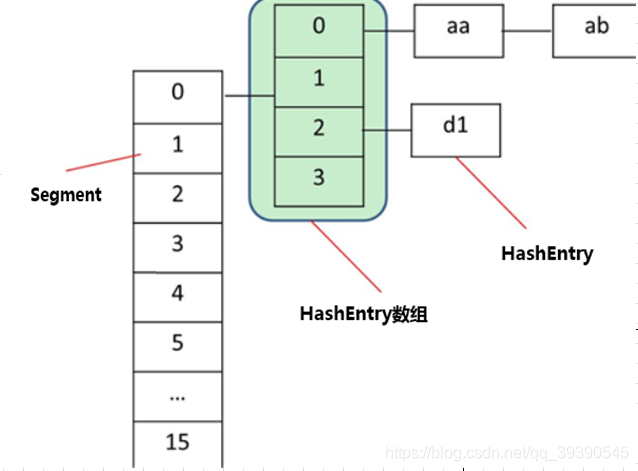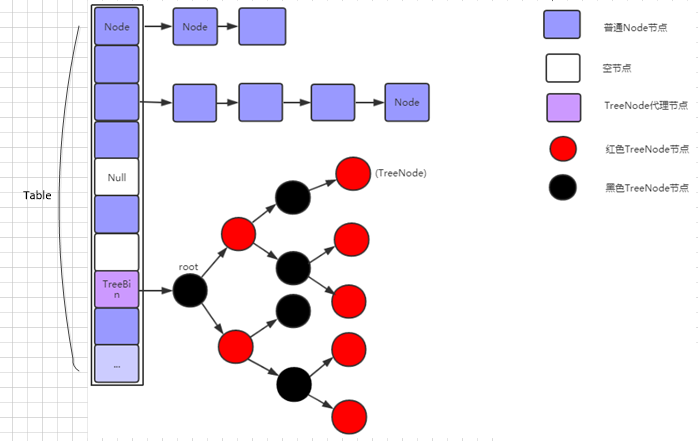Java 岗大厂面试百日冲刺 - 日积月累，每日三题【Day19】—

• 2021 年 11 月 11 日
• 本文字数：5709 字

阅读完需：约 19 分钟

JDK1.8 为什么使用内置锁 synchronized 来代替重入锁 ReentrantLock，主要有以下几点：

1. 因为粒度降低了，在相对而言的低粒度加锁方式，synchronized 并不比 ReentrantLock 差，在粗粒度加锁中 ReentrantLock 可能通过 Condition 来控制各个低粒度的边界，更加的灵活，而在低粒度中，Condition 的优势就没有了

2. JVM 的开发团队从来都没有放弃 synchronized，而且基于 JVM 的 synchronized 优化空间更大，使用内嵌的关键字比使用 API 更加自然

3. 在大量的数据操作下，对于 JVM 的内存压力，基于 API 的 ReentrantLock 会开销更多的内存，虽然不是瓶颈，但是也是一个原因之一。

JDK1.7 版本的 get putSegment 数组的意义就是将一个大的 table 分割成多个小的 table 来进行加锁，也就是上面的提到的锁分段技术，而每一个Segment元素存储的HashEntry数组+链表，这个和 HashMap 的数据存储结构一样。

【一线大厂Java面试题解析+后端开发学习笔记+最新架构讲解视频+实战项目源码讲义】

ConcurrentHashMap 的初始化是会通过位与运算来初始化 Segment 的大小，用 ssize 来表示，源码如下所示

private static final int DEFAULT_CONCURRENCY_LEVEL = 16;

private void writeObject(java.io.ObjectOutputStream s)

throws java.io.IOException {

// For serialization compatibility

// Emulate segment calculation from previous version of this class

int sshift = 0;

int ssize = 1;

while (ssize < DEFAULT_CONCURRENCY_LEVEL) {

++sshift;

ssize <<= 1;

}

int segmentShift = 32 - sshift;

int segmentMask = ssize - 1;

int cap = 1;

while (cap < c)

cap <<= 1

JDK1.7 —— put 操作

static class Segment<K,V> extends ReentrantLock implements Serializable {

private static final long serialVersionUID = 2249069246763182397L;

Segment(float lf) { this.loadFactor = lf; }

}

JDK1.7 —— get 操作

ConcurrentHashMap 的 get 操作跟 HashMap 类似，只是 ConcurrentHashMap第一次需要经过一次hash定位到Segment的位置然后再hash定位到指定的HashEntry，遍历该 HashEntry 下的链表进行对比，成功就返回，不成功就返回 nullJDK1.8 版本的 get put

• 改进一：取消segments字段，直接采用transient volatile HashEntry<K,V>[] table保存数据，采用 table 数组元素作为锁，从而实现了对每一行数据进行加锁，进一步减少并发冲突的概率。

• 改进二：将原先 table 数组＋单向链表的数据结构，变更为 table 数组＋单向链表＋红黑树的结构。

??对于 hash 表来说，最核心的能力在于将 key hash 之后能均匀的分布在数组中。如果hash之后散列的很均匀，那么 table 数组中的每个队列长度基本都为0或者1才对

??但实际情况并非总是如此理想，虽然 ConcurrentHashMap 类默认的加载因子为 0.75，但是在数据量过大或者运气不佳的情况下，还是会存在一些队列长度过长的情况，如果还是采用单向列表方式，那么查询某个节点的时间复杂度为O(n)

??因此，对于个数超过 8(默认值)的列表，jdk1.8 中采用了红黑树的结构，那么查询的时间复杂度可以降低到 O(logN)，从而针对该种情况，改进了性能。

JDK1.8的实现已经摒弃了Segment的概念，而是直接用Node数组+链表+红黑树的数据结构来实现，并发控制使用 Synchronized 和 CAS 来操作，整个看起来就像是优化过且线程安全的 HashMap，虽然在 JDK1.8 中还能看到 Segment 的数据结构，但是已经简化了属性，只是为了兼容旧版本。

// node 数组最大容量：2^30=1073741824

private static final int MAXIMUM_CAPACITY = 1 << 30;

// 默认初始值，必须是 2 的幕数

private static final int DEFAULT_CAPACITY = 16

//数组可能最大值，需要与 toArray（）相关方法关联

static final int MAX_ARRAY_SIZE = Integer.MAX_VALUE - 8;

//并发级别，遗留下来的，为兼容以前的版本

private static final int DEFAULT_CONCURRENCY_LEVEL = 16;

// 负载因子

private static final float LOAD_FACTOR = 0.75f;

// 链表转红黑树阀值,> 8 链表转换为红黑树

static final int TREEIFY_THRESHOLD = 8;

//树转链表阀值，小于等于 6（tranfer 时，lc、hc=0 两个计数器分别++记录原 bin、新 binTreeNode 数量，<=UNTREEIFY_THRESHOLD 则 untreeify(lo)）

static final int UNTREEIFY_THRESHOLD = 6;

static final int MIN_TREEIFY_CAPACITY = 64;

private static final int MIN_TRANSFER_STRIDE = 16;

private static int RESIZE_STAMP_BITS = 16;

// 2^15-1，help resize 的最大线程数

private static final int MAX_RESIZERS = (1 << (32 - RESIZE_STAMP_BITS)) - 1;

// 32-16=16，sizeCtl 中记录 size 大小的偏移量

private static final int RESIZE_STAMP_SHIFT = 32 - RESIZE_STAMP_BITS;

// forwarding nodes 的 hash 值

static final int MOVED = -1;

// 树根节点的 hash 值

static final int TREEBIN = -2;

// ReservationNode 的 hash 值

static final int RESERVED = -3;

// 可用处理器数量

static final int NCPU = Runtime.getRuntime().availableProcessors();

//存放 node 的数组

transient volatile Node<K,V>[] table;

/*控制标识符，用来控制 table 的初始化和扩容的操作，不同的值有不同的含义

*当为负数时：-1 代表正在初始化，-N 代表有 N-1 个线程正在 进行扩容

*当为 0 时：代表当时的 table 还没有被初始化• Node

HashEntry == Node

Node 是 ConcurrentHashMap 存储结构的基本单元，继承于 HashMap 中的 Entry，用于存储数据，Node就是一个链表，但是只允许对数据进行查找，不允许进行修改;

• TreeNode

TreeNode 继承与 Node，但是数据结构换成了二叉树结构，它是红黑树的数据的存储结构，用于红黑树中存储数据，当链表的节点数大于 8 时会转换成红黑树的结构，他就是通过 TreeNode 作为存储结构代替 Node 来转换成黑红树。源代码如下

• TreeBin

TreeBin 从字面含义中可以理解为存储树形结构的容器，而树形结构就是指 TreeNode，所以 TreeBin 就是封装 TreeNode 的容器，它提供转换黑红树的一些条件和锁的控制。

public class TestConcurrentHashMap{

public static void main(String[] args){

ConcurrentHashMap<String,String> map = new ConcurrentHashMap(); //初始化 ConcurrentHashMap

//新增个人信息

map.put("id","1");

map.put("name","andy");

map.put("sex","男");

//获取姓名

String name = map.get("name");

Assert.assertEquals(name,"andy");

//计算大小

int size = map.size();

Assert.assertEquals(size,3);

}

}

public ConcurrentHashMap() {

}

JDK1.8 —— put 操作

public V put(K key, V value) {

return putVal(key, value, false);

}

/** Implementation for put and putIfAbsent */

final V putVal(K key, V value, boolean onlyIfAbsent) {

if (key == null || value == null) throw new NullPointerException();

int hash = spread(key.hashCode()); //两次 hash，减少 hash 冲突，可以均匀分布

int binCount = 0;

for (Node<K,V>[] tab = table;;) { //对这个 table 进行迭代

Node<K,V> f; int n, i, fh;

//这里就是上面构造方法没有进行初始化，在这里进行判断，为 null 就调用 initTable 进行初始化，属于懒汉模式初始化

if (tab == null || (n = tab.length) == 0)

tab = initTable();

else if ((f = tabAt(tab, i = (n - 1) & hash)) == null) {//如果 i 位置没有数据，就直接无锁插入

if (casTabAt(tab, i, null,

new Node<K,V>(hash, key, value, null)))

break; // no lock when adding to empty bin

}

else if ((fh = f.hash) == MOVED)//如果在进行扩容，则先进行扩容操作

tab = helpTransfer(tab, f);

else {

V oldVal = null;

//如果以上条件都不满足，那就要进行加锁操作，也就是存在 hash 冲突，锁住链表或者红黑树的头结点

synchronized (f) {

if (tabAt(tab, i) == f) {

if (fh >= 0) { //表示该节点是链表结构

binCount = 1;

for (Node<K,V> e = f;; ++binCount) {

K ek;

//这里涉及到相同的 key 进行 put 就会覆盖原先的 value

if (e.hash == hash &&

((ek = e.key) == key ||

(ek != null && key.equals(ek)))) {

oldVal = e.val;

if (!onlyIfAbsent)

e.val = value;

break;

}

Node<K,V> pred = e;

if ((e = e.next) == null) { //插入链表尾部

pred.next = new Node<K,V>(hash, key,

value, null);

break;

}

}

}

else if (f instanceof TreeBin) {//红黑树结构

Node<K,V> p;

binCount = 2;

//红黑树结构旋转插入

if ((p = ((TreeBin<K,V>)f).putTreeVal(hash, key,

value)) != null) {

oldVal = p.val;

if (!onlyIfAbsent)

p.val = value;

}

}

}

}

if (binCount != 0) { //如果链表的长度大于 8 时就会进行红黑树的转换

if (binCount >= TREEIFY_THRESHOLD)

treeifyBin(tab, i);

if (oldVal != null)

return oldVal;

break;

}

}

}

return null;

}

1. 如果没有初始化就先调用initTable()方法来进行初始化过程

2. 如果没有 hash 冲突就直接 CAS 插入

3. 如果还在进行扩容操作就先进行扩容

4. 如果存在 hash 冲突，就加锁来保证线程安全，这里有两种情况，一种是链表形式就直接遍历到尾端插入一种是红黑树就按照红黑树结构插入

5. 最后一个如果该链表的数量大于阈值 8，就要先转换成黑红树的结构，break 再一次进入循环，默认的链表大小，超过了这个值就会转换为红黑树;

put 的流程你可以从中发现，他在并发处理中使用的是乐观锁，当有冲突的时候才进行并发处理

JDK1.8 —— get 操作

public V get(Object key) {

Node<K,V>[] tab; Node<K,V> e, p; int n, eh; K ek;

int h = spread(key.hashCode()); //计算两次 hash

if ((tab = table) != null && (n = tab.length) > 0 &&

(e = tabAt(tab, (n - 1) & h)) != null) {//读取首节点的 Node 元素

if ((eh = e.hash) == h) { //如果该节点就是首节点就返回

if ((ek = e.key) == key || (ek != null && key.equals(ek)))

return e.val;

}

//hash 值为负值表示正在扩容，这个时候查的是 ForwardingNode 的 find 方法来定位到 nextTable 来

//查找，查找到就返回

else if (eh < 0)

return (p = e.find(h, key)) != null ? p.val : null;

while ((e = e.next) != null) {//既不是首节点也不是 ForwardingNode，那就往下遍历

if (e.hash == h &&

((ek = e.key) == key || (ek != null && key.equals(ek))))

return e.val;

}

}

return null;

}

ConcurrentHashMap 的 get 操作的流程很简单，也很清晰，可以分为三个步骤来描述

1. 计算 hash 值，定位到该table索引位置，如果是首节点符合就返回

2. 如果遇到扩容的时候，会调用标志正在扩容节点 ForwardingNode 的 find 方法，查找该节点，匹配就返回

3. 以上都不符合的话，就往下遍历节点，匹配就返回，否则最后就返回null

get 方法不需要加锁。因为 Node 的元素 value 和指针 next 是用 volatile 修饰的（可见性），在多线程环境下线程A修改节点的 value 或者新增节点的时候是对线程B可见的评论